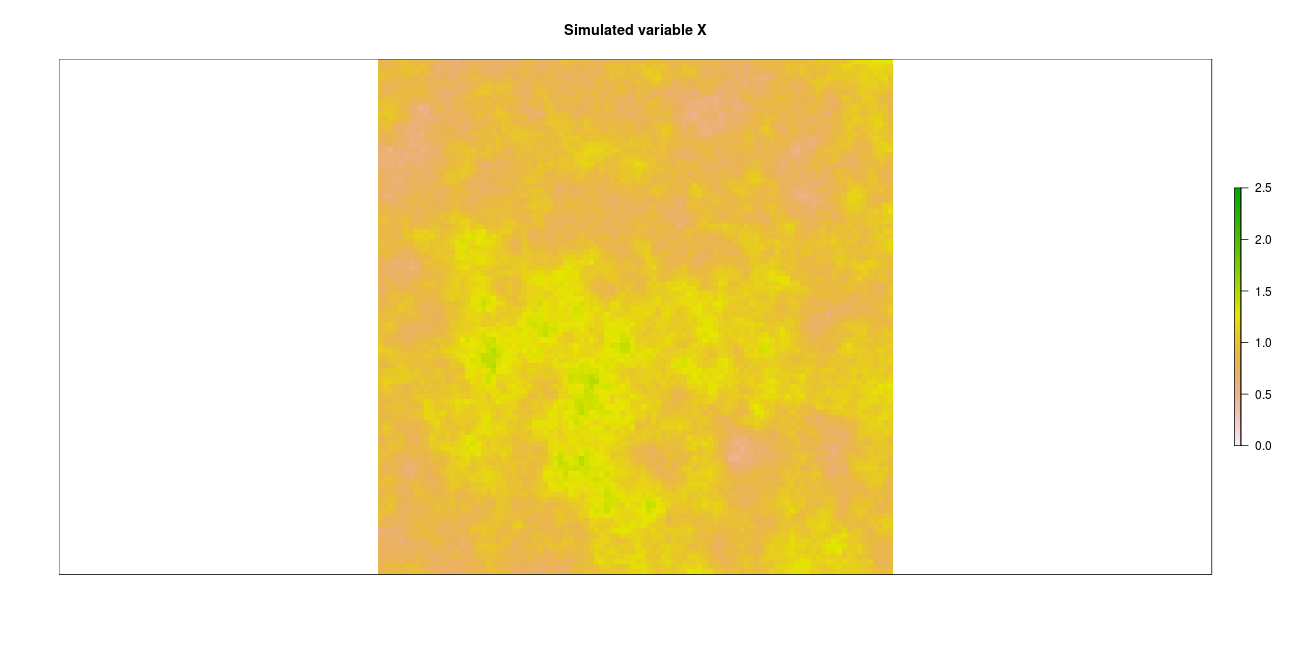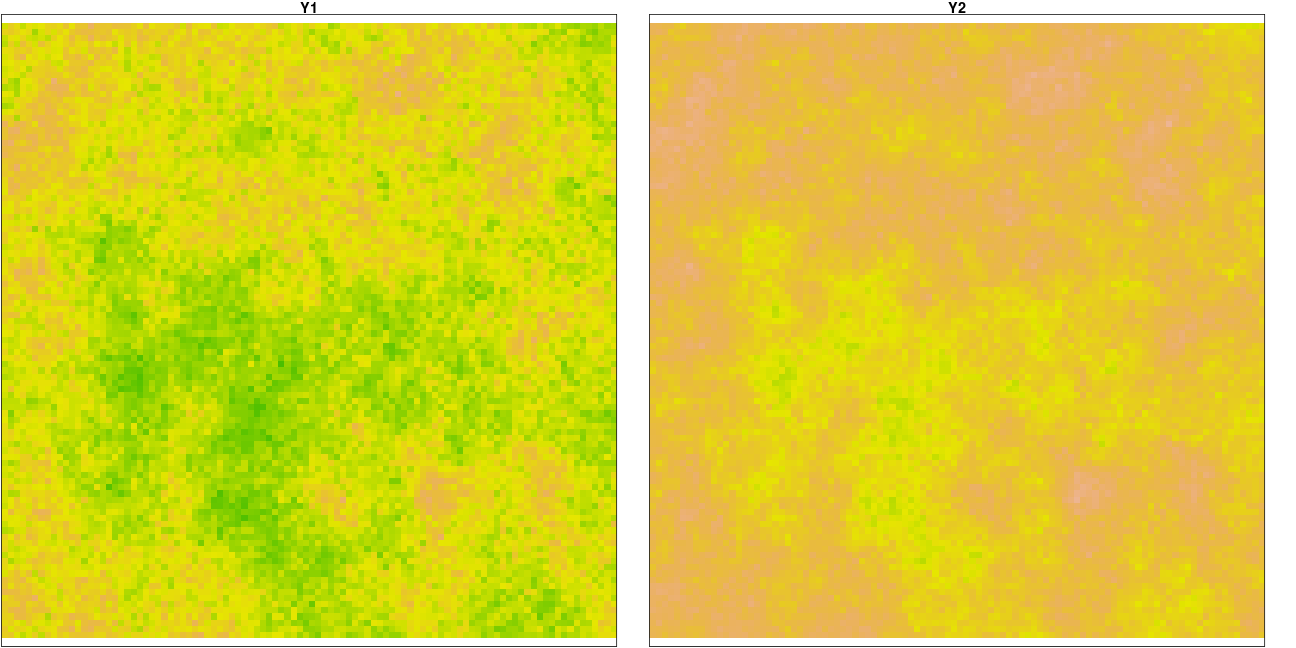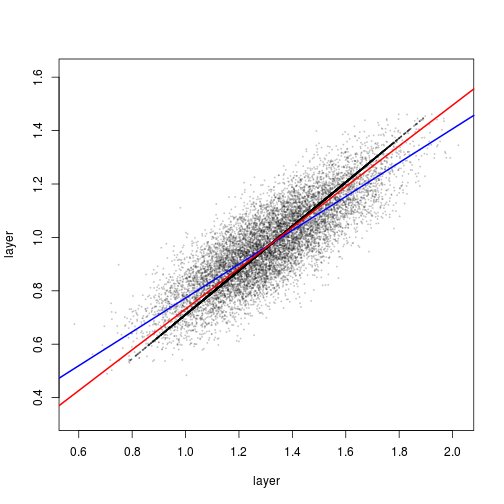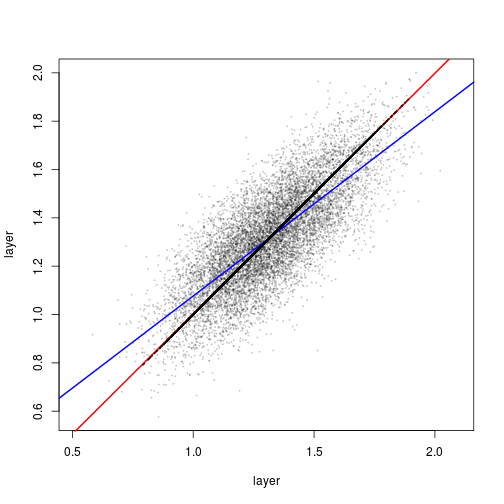# Goal

The evaluation of a satellite product normally includes exploring its consistency with respect to former products. For this comparison to be considered satisfactory, it should be possible to describe the given relationship by an spatially-independent simple model, best a linear model. Parameters of the model must be estimated taking into account that both variables are subject to error. Spatial independence implies that the same model links both products in different geographic regions, and the statistical method to proof it involves exploring the spatial distribution of the residuals and testing for spatial autocorrelation.
Here I present an example with artificial data. As we control the way we generate them, artificial data allows us to verify that our conclusions are actually correct.

## 1. Simulation of geographic fields

### 1.1 Simulation of a variable field

We simulate a geographic field of a surface variable (i.e. LAI) X as a random field with autocorrelation defined by an exponential variogram.### 1.2. Simulation of satellite products

Let’s assume we have two satellite products estimating the field of the surface variable X: a former (i.e. MODIS MOD15) and a new product (i.e. GEOv3 LAI), named Y1 and Y2 respectively, for which we assume the favourable case of linear relationships with the actual variable. Note we make Y2 to be a more accurate estimate of X than Y1:

`y1 = 1.15x + 0.20 + N(0,0.10)`
`y2 = 0.95x + 0.05 + N(0,0.05)`## 2. Comparing the two satellite products

We now pretend we do not know the above functions and investigate the relationship between Y1 and Y2 through a linear model: `Y2 = a + bY1 + e`
We must estimate the parameters and explore the spatial structure of e.

## 1.1 Estimating the model parameters

``````##
## Model II regression
##
## Call: lmodel2(formula = y2[] ~ y1[])
##
## n = 10000   r = 0.8298143   r-square = 0.6885918
## Parametric P-values:   2-tailed = 0    1-tailed = 0
## Angle between the two OLS regression lines = 10.26006 degrees
##
## Regression results
##   Method   Intercept     Slope  Angle (degrees)  P-perm (1-tailed)
## 1    OLS  0.13921251 0.6336102         32.35876                 NA
## 2     MA  0.02059837 0.7236945         35.89305                 NA
## 3    SMA -0.03188801 0.7635566         37.36378                 NA
##
## Confidence intervals
##   Method  2.5%-Intercept 97.5%-Intercept  2.5%-Slope 97.5%-Slope
## 1    OLS     0.128079318      0.15034569   0.6252570   0.6419633
## 2     MA     0.007978597      0.03310429   0.7141966   0.7332789
## 3    SMA    -0.042946770     -0.02094957   0.7552491   0.7719554
##
## Eigenvalues: 0.06227036 0.005314434
##
## H statistic used for computing C.I. of MA: 3.920566e-05``````There are two important facts to be highlighted:

• The added noise changes the slope of the noise-free relationship, represented by the black line and with slope equal to the ratio of slopes vs. X: 0.95/1.15 = 0.826.
• The Ordinary Least Squares regression (OLS, blue line) calculates a significantly lower slope than that of the main axis of the cloud, which is estimated by the Standardized Major Axis regression (SMA, red line). We will pay special attention to this fact in the next section.

## 2.2 OLS vs Major Axis methods

In this section we highlight the consequences of the method that is used to estimate the model parameters when both variables have noise. In order to put the impact of the choice in relevance, we now assume we have a second version of the first product that just differs on having a different realization of the random noise but has identical parameters:

`y1 = 1.15x + 0.20 + N(0,0.20)`
`y1b = 1.15x + 0.20 + N'(0,0.20)`

Note that we have made, by construction, both products to be related by an slope of 1.

``````##
## Model II regression
##
## Call: lmodel2(formula = y1b[] ~ y1[])
##
## n = 10000   r = 0.7656634   r-square = 0.5862404
## Parametric P-values:   2-tailed = 0    1-tailed = 0
## Angle between the two OLS regression lines = 15.11994 degrees
##
## Regression results
##   Method   Intercept     Slope  Angle (degrees)  P-perm (1-tailed)
## 1    OLS 0.314311038 0.7622769         37.31744                 NA
## 2     MA 0.008901695 0.9942274         44.83415                 NA
## 3    SMA 0.007124508 0.9955771         44.87301                 NA
##
## Confidence intervals
##   Method  2.5%-Intercept 97.5%-Intercept  2.5%-Slope 97.5%-Slope
## 1    OLS     0.297578484      0.33104359   0.7497226   0.7748312
## 2     MA    -0.012837235      0.03028755   0.9779854   1.0107375
## 3    SMA    -0.009509989      0.02355057   0.9831020   1.0082106
##
## Eigenvalues: 0.07505011 0.009960301
##
## H statistic used for computing C.I. of MA: 6.780879e-05``````The OLS method estimates an slope (blue line) that is significantly different (and lower) than 1, while both MA and SMA #’ correctely retrieve an slope that is not significantly different from 1 (not only the values of the slopes are very close to 1, but their 5% confidence interval includes 1).
The lower OLS slope is a consequence of the OLS method assuming all the dispersion is due to the dependent variable only, which is not the case when comparing two products. OLS minimizes the squared differences between the observed Y and the predicted Y. MA and SMA take the dispersion in both variables into consideration: MA minimizes the perpendicular distance from the observed point to the predicted line, and SMA minimizes the area of the triangle defined by the observation and the corresponding values on the predicted line. SMA is a more appropriate method when the dispersion of the variables being regressed is different.

## 2.3 Spatial independence

Spatial independence is important because implies that the same model links both products in different geographic regions. The statistical method to proof it involves exploring the spatial distribution of the residuals and testing for spatial autocorrelation. It is important to note that the spatial distribution of the residuals is not the same as the spatial distribution of the differences, except in the case of a linear relationship with slope of 1. For example, we define

`Y4 = a + bY3 + e`
`Y3 = 10.2 + 1.35x + N(0,0.15)`
`Y4 = 0.1 + 3.10x + N(0,0.10)`

and estimate the model

``````##
## Model II regression
##
## Call: lmodel2(formula = y2c[] ~ y1c[])
##
## n = 10000   r = 0.8025217   r-square = 0.6440412
## Parametric P-values:   2-tailed = 0    1-tailed = 0
## Angle between the two OLS regression lines = 10.30023 degrees
##
## Regression results
##   Method Intercept     Slope  Angle (degrees)  P-perm (1-tailed)
## 1    OLS -14.62206  1.540633         57.01305                 NA
## 2     MA -22.18874  2.198008         65.53649                 NA
## 3    SMA -18.98575  1.919740         62.48482                 NA
##
## Confidence intervals
##   Method  2.5%-Intercept 97.5%-Intercept  2.5%-Slope 97.5%-Slope
## 1    OLS       -14.88058       -14.36354    1.518179    1.563087
## 2     MA       -22.56198       -21.82440    2.166355    2.230434
## 3    SMA       -19.24571       -18.72881    1.897418    1.942325
##
## Eigenvalues: 0.2970287 0.02025264
##
## H statistic used for computing C.I. of MA: 3.017944e-05``````

and we plot Differences and Residuals